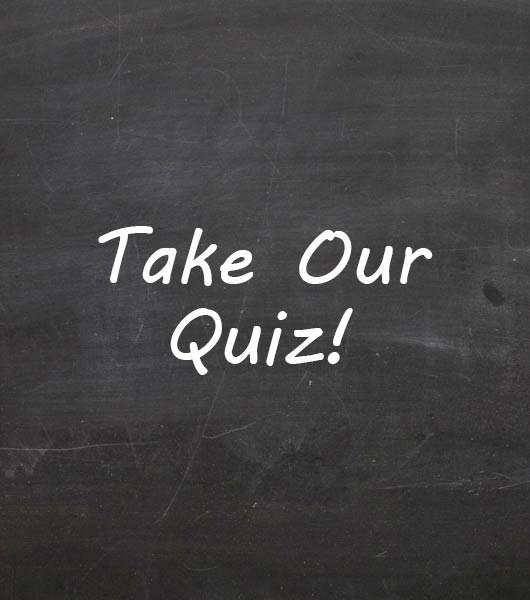Newton's Law Assessment Noel Pd 2

34 Questions | Total Attempts: 32Settings• 1.
In a head-on collision, any passenger wearing a seat belt becomes "part" of the car.
• A.

True

• B.

False

• 2.
If a net or unbalanced force acts on an object, the object will change speed, and or directions
• A.

True

• B.

False

• 3.
Gravity is the tendency of  an object to resist any change in its motion.
• A.

True

• B.

False

• 4.
A force is a push or pull that one body exerts on another body.
• A.

True

• B.

False

• 5.
Friction is the force that increase motion between two surfaces that are touching each other.
• A.

True

• B.

False

• 6.
The greater an object's mass, the weaker the gravitational force on it.
• A.

True

• B.

False

• 7.
A person in a head-on collision, who is not wearing a seat belt, continues to move forward at the same speed of the car because of _____.
• A.

Inertia

• B.

Friction

• C.

Weigth

• D.

Gravity

• 8.
Forces that are equal in size and opposite in direction are _____.
• A.

Unbalanced/net forces

• B.

Frictional Forces

• C.

Balanced Forces

• D.

Gravitational Forces

• 9.
Newton's first law of motion states that an object at rest stays at rest unless a(n) ____ acts on it.
• A.

Balanced Force

• B.

Unbalanced/Net Force

• C.

Weak Force

• D.

Strong Force

• 10.
At the same speed, which object has the greatest momentum?
• A.

Bowling Ball

• B.

Ping Pong Ball

• C.

Golf Ball

• D.

Marble

• 11.
The amount of gravitational force between objects depends on their ____.
• A.

Frictional Forces

• B.

Speed and Direction

• C.

Intertia

• D.

The object's masses and the distance between them

• 12.
The correct value and units for momentum are ________.
• A.

50 kg

• B.

-20 m/s/s

• C.

100 kg x m/s

• D.

112 N

• E.

20 m/s/s

• 13.
The correct value and units for acceleration are ________.
• A.

50 kg

• B.

-20 m/s/s

• C.

100 kg x m/s

• D.

112 N

• E.

30 m/s North

• 14.
The correct value and units for weight or force are ________.
• A.

50 kg

• B.

-20 m/s/s

• C.

100 kg x m/s

• D.

112 N

• E.

20 m/s/s

• 15.
The correct value and units for velocity are ________.
• A.

50 kg

• B.

-20 m/s/s

• C.

100 kg x m/s

• D.

112 N

• E.

30 m/s North

• 16.
The upward force exerted on an object falling though air is ______.
• A.

Terminal Velocity

• B.

Momentum

• C.

Air Resistance

• D.

Weightlessness

• 17.
Friction always act in a direction ___ to the object's direction of motion
• A.

Equal

• B.

Opposite

• C.

Perpendicular

• D.

Parallel

• 18.
In the equation, p=mv, p reprents ____
• A.

Momentum

• B.

Friction

• C.

Interia

• D.

Velocity

• 19.
The relationship among force, mass and acceleration is explained by ____.
• A.

Newton's First Law of Motion

• B.

Newton's Second Law of Motion

• C.

Newton's Third Law of Motion

• D.

The Law of Conservation of Momentum

• 20.
When a gun is fired the "kick back" of the gun is explained by ______.
• A.

Newton's First Law of Motion

• B.

Newton's Second Law of Motion

• C.

Newton's Third Law of Motion

• D.

The Law of Inertia

• 21.
A feather will fall through the air more slowly than a brick because of ____
• A.

Gravity

• B.

Air Resistance

• C.

Terminal Velocity

• D.

Inertia

• 22.
In the absence of air, a penny and a feather that are dropped from the same height will_____.
• A.

Fall at different rates

• B.

Float

• C.

Fall at the same rates

• D.

Not have momentum

• 23.
If a 300 N action force is exerted to the right, the reaction force will be ______.
• A.

300 N to the right

• B.

600 N to the right

• C.

300 N to the left

• D.

600 N to the left

• 24.
Acceleration due to gravity is _____.
• A.

98 m/s/s

• B.

9.8 m/s/s

• C.

9.8 m/s

• D.

.98 m/s

• 25.
A real car moving at 10 km/hr has more momentum than a toy car moving at the same rate because ______.
• A.

The real car has a greater mass

• B.

The real car has less mass

• C.

The real car moves faster

• D.

Of frictionBack to top